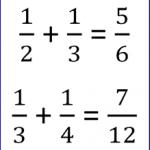Smartick is a fun way to learn math!# All posts by Smartick

Content Creation Team. A multidisciplinary and multicultural team made up of mathematicians, teachers, professors and other education professionals! They strive to create the best math content possible.

Jun22

## Rekenrek Vs. Soroban I: Hand AnalogyRekenrek Vs. Soroban At Smartick, we use the abacus as a visual model to learn how to calculate. Some other well-known methods, like Aloha or UCMAS, use the traditional Japanese abacus, the Soroban, as a means to reach the same goal. We’ve opted for a different kind of abacus: the Rekenrek. The Rekenrek is an abacus […]

Jun16

## How to Solve Double Digit Division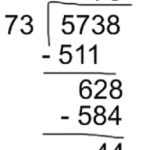In today’s post we are going to explain how to solve double digit division. Before beginning to learn how to solve double digit division, it is important that you become familiar with these terms, because we will use them later. Dividend: the number that is being divided. Divisor: the number by which the dividend is […]

Jun15

## Geometric Shape: Properties of a SquareToday, we’re going to take a look at a shape that you definitely know already, but maybe you aren’t familiar with all of its main characteristics. I’m talking about the square. Geometric Shape: Square The square is a geometric shape that belongs to the quadrilateral family because it has 4 sides. The 4 sides are […]

Jun10

## Dividing a Decimal by a Whole Number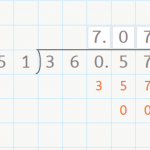Dividing a Decimal by a Whole Number Hello everyone! In this week’s post, we are going to talk about dividing a decimal by a whole number. You already know how to divide a whole number by a whole number by now, right? If not, check out our posts about dividing by a one-digit number here, […]

Jun09

## Properties of Multiplication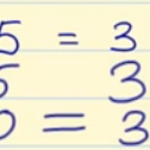The properties of multiplication are distributive, commutative, associative, removing a common factor and the neutral element. We are dedicating this post to examining the properties of multiplication, which are the following: Distributive property: The multiplication of a number by a sum is equal to the sum of the multiplications of this number by each one […]

Jun08

## Learn How to Subtract without Regrouping

Hello and welcome back! Today we are going to learn how to subtract without regrouping. Are you ready? Subtract Without Regrouping We’re going to follow a few steps. If you follow them well, you’ll learn how to subtract without regrouping in no time: You need to place the subtrahend below the minuend so that the ones-place […]

Jun03

## Solve and Analyze Numerical Place Values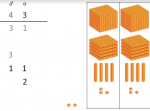Numerical Place Values What are the numerical place values? Why does a number’s value change according to its position? We’ll explain everything you need to know here about understanding mathematical algorithms by working with numerical place values. In this post, we are going to learn what numerical place value is, why it’s important and how […]